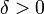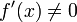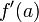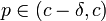# First derivative test is conclusive for differentiable function at isolated critical point

This article describes a situation, or broad range of situations, where a particular test or criterion is conclusive, i.e., it works as intended to help us determine what we would like to determine.
The test is first derivative test. See more conclusive cases for first derivative test | inconclusive cases for first derivative test

## Statement

Suppose$f$ is a function and$c$ is a point in the interior of the domain of$f$. Suppose the following hold:

•$c$ is a critical point for$f$.
•$f$ is continuous at$c$.
•$f$ is differentiable on the immediate left and immediate right of$c$.
•$c$ is an isolated critical point for$f$, i.e., there is an open interval containing$c$ in which it is the only critical point for$f$.

Then, the first derivative test is conclusive at$c$, i.e., we can use the first derivative test to conclusively determine whether$f$ has a local maximum, local minimum, or neither at$c$.

## Related facts

### Motivating opposite fact

The isolated critical point condition is to avoid the scenario of first derivative test is inconclusive for function whose derivative has ambiguous sign around the point.

## Facts used

1. Derivative of differentiable function satisfies intermediate value property: Note that if we assume$f$ to be continuously differentiable on the immediate left and right of$c$, we can use the intermediate value theorem instead to show that the derivative$f'$ is continuous on the immediate left and immediate right of$c$.

## Proof

### Proof idea

The key idea behind the proof is to show that, to the immediate left of$c$,$f$ must have constant sign, i.e., it must be either positive everywhere or negative everywhere on the immediate left of$c$. Similarly, we show that, to the immediate right of$c$,$f$ must have constant sign.

We can then use the first derivative test without ambiguity.

### Proof details

Given:$f$ is a function and$c$ is a point in the interior of the domain of$f$ such that:

•$c$ is a critical point for$f$.
•$f$ is continuous at$c$.
•$f$ is differentiable on the immediate left and immediate right of$c$.
•$c$ is an isolated critical point for$f$, i.e., there is an open interval containing$c$ in which it is the only critical point for$f$.

To prove:$f'$ has constant sign to the immediate left of$c$. Also,$f'$ has constant sign to the immediate right of$c$ (note that these constant signs may be the same or different from each other). Thus, the first derivative test is conclusive.

Proof: We provide a proof for the immediate left. A similar proof works for the immediate right.

Step no. Assertion/construction Facts used Given data used Previous steps used Explanation
1$f'$ is defined and nonzero on the immediate left of$c$. In other words, there exists$\delta > 0$ such that$f'(x) \ne 0$ for$x \in (c - \delta,c)$.$c$ is an isolated critical point for$f$. Direct from given.
2 Suppose there exist$a < b$, such that both$a,b$ are in$(c - \delta,c)$ such that$f'(a)$ and$f'(b)$ have opposite signs. Then, there exists$p \in (a,b)$ such that$f'(p) = 0$. In particular,$p \in (c - \delta, c)$. Fact (1) Direct from fact, and the observation that 0 lies between any positive and negative number.
3$f'$ must have constant sign (i.e., positive throughout or negative throughout) on$(c - \delta, c)$ Steps (1), (2) Step-combination direct